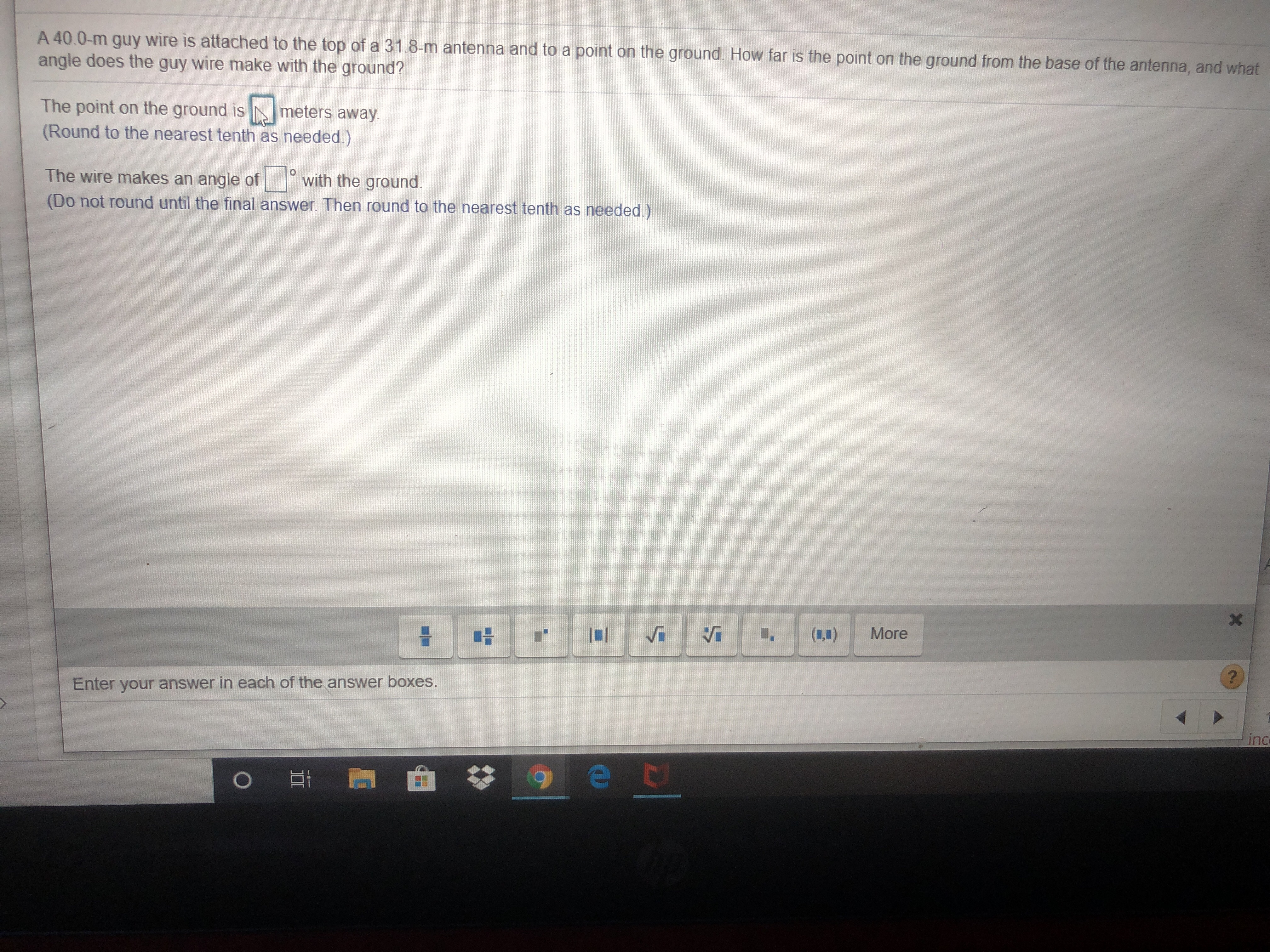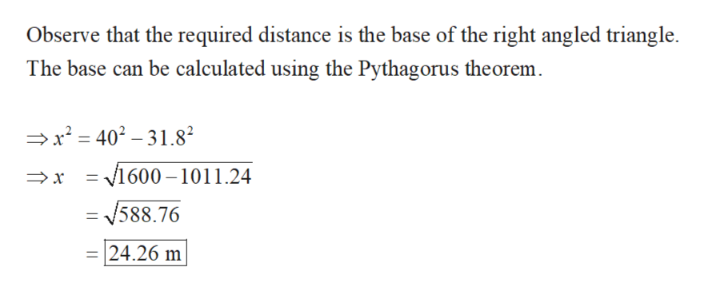A 40.0-m guy wire is attached to the top of a 31.8-m antenna and to a point on the ground. How far is the point on the ground from the base of the antenna, and whatangle does the guy wire make with the ground?The point on the ground is(Round to the nearest tenth as needed.)meters away.The wire makes an angle ofwith the ground(Do not round until the final answer. Then round to the nearest tenth as needed.)XMore(40)Enter your answer in each of the answer boxes.ince t

Question

Please help. I’ve done this question 3 times and got it wrong. I don’t know what I’m doing wrong.

It reads: a 40.0 guy wire isn’t attached to the he top of a 31.8-m antenna and to a point on a ground. How far Is the point on the ground from the base of the antenna, and what angle does the guy wire make with the ground?

The point on the  ground is ___ meters away.

The wire makes an angle of ___ degrees with the ground.help_outlineImage TranscriptioncloseA 40.0-m guy wire is attached to the top of a 31.8-m antenna and to a point on the ground. How far is the point on the ground from the base of the antenna, and what angle does the guy wire make with the ground? The point on the ground is (Round to the nearest tenth as needed.) meters away. The wire makes an angle of with the ground (Do not round until the final answer. Then round to the nearest tenth as needed.) X More (40) Enter your answer in each of the answer boxes. inc e t fullscreen
Step 1

The given situation can be inscribed in a right angled triangle as follows.

Step 2

Obtain the distance between the base of the anten...help_outlineImage TranscriptioncloseObserve that the required distance is the base of the right angled triangle The base can be calculated using the Pythagorus theorem x2= 401.8 = 1600-1011.24 /588.76 24.26 m fullscreen

Want to see the full answer?

See Solution

Want to see this answer and more?

Our solutions are written by experts, many with advanced degrees, and available 24/7

See Solution
Tagged in

Trigonometric Ratios